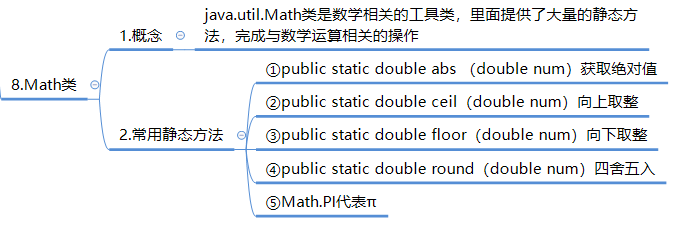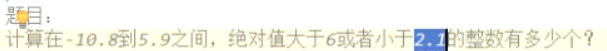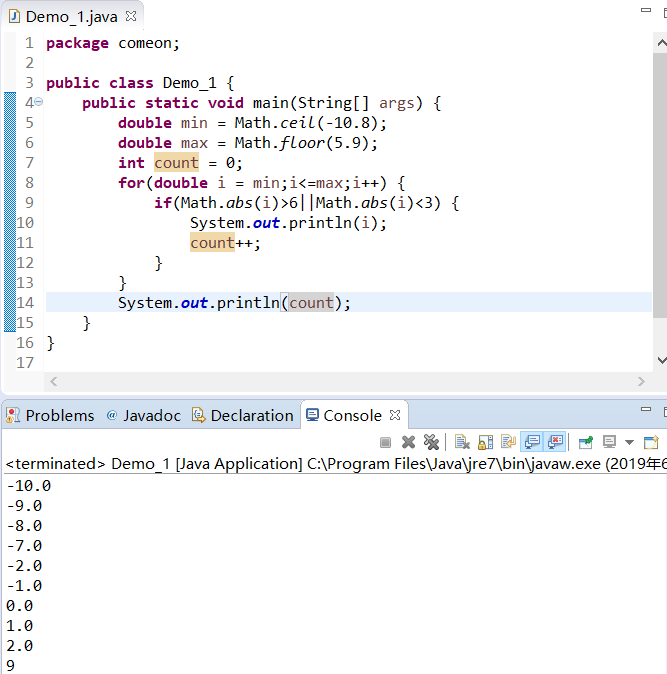• Java为开发者提供了基本的数学工具类——Math类。Math类包含用于执行基本数学运算的方法，如初等指数、对数、平方根和三角函数。本实验我们将利用这些方法，对数值进行复杂的运算。 Java数学工具包java.lang.Math类...
Java为开发者提供了基本的数学工具类——Math类。Math类包含用于执行基本数学运算的方法，如初等指数、对数、平方根和三角函数。本实验我们将利用这些方法，对数值进行复杂的运算。 Java数学工具包java.lang.Math类 Java SE官方API文档 - Java.lang.Math Java SE官方API文档 - Java.lang.Random

1. Math类中的常量

Math类自带了两个常量，分别是：

比任何其他值都更接近 e（即自然对数的底数）的 double 值。
比任何其他值都更接近 pi（即圆的周长与直径之比）的 double 值。

public class ShowConstant {

public static void main(String[] args) {

System.out.println("The natural logarithm is " + Math.E);
System.out.println("The circumference ratio is " + Math.PI);
}
}

2. 如何利用数学工具

2.1 三角函数计算

public class TriFunction {
public static void main(String[] args)
{
System.out.println("sin30 = "+Math.sin(Math.PI/6));
System.out.println("cos30 = " + Math.cos(Math.PI / 6));
System.out.println("tan30 = " + Math.tan(Math.PI / 6));
}

}


sin30 = 0.49999999999999994
cos30 = 0.8660254037844387
tan30 = 0.5773502691896257

2.2 生成彩票号码

目前市面上彩票的种类很多，我们就以大乐透为例吧。大乐透的规则是：从1~35中随机选取5个不重复的数字，从1~12中随机选取2个不重复的数字，这些数字组成了一个七位数。如果你买的号码与摇出来的号码相同，那么你一夜之间可能就不会当程序员了。

对于该项目所需要用到的API，你需要引入下面一些包：

import java.util.ArrayList;
import java.util.Collections;
import java.util.List;
import java.util.Random;

由于彩票号码的前半段和后半段的规则和个数略有不同，所以我们分别创建生成它们的首先来创建生成前段号码的方法getHeadNumber()，该方法需要从1到36中随机选取一个数作为号码（即摇号的过程）。对于个位数，为了显示上更整齐，所以我们在其十位再放一个“0”。再者，为了结果更加易读，我们还需要调用Collections.sort()方法对结果进行排序。

代码片段如下：

0) {
list.add("0" + i + "  ");
// 在集合中添加0~9的号码，因为是个位数，为了显示上更加人性化，所以需要在十位的地方添加“0”
} else {
list.add("" + i + "  ");
// 向集合添加大于9的号码，即添加双位数
}
}

int roundIndex = 0;
// 创建并初始化随机数

List<String> lotteryList = new ArrayList<String>();
// 保存前段号码的List集合

for (int j = 0; j < 5; j++) {
int amount = list.size(); // 获取前半段号码的个数
Random r = new Random(); // 创建并实例化Random的对象
roundIndex = r.nextInt(amount); // 获取一个0到amount-1的随机数
lotteryNumber = list.get(roundIndex); // 获取彩票数字，即摇号的号码
lotteryList.add(lotteryNumber); // 将彩票号码添加lotteryList中
list.remove(roundIndex); // 移除刚刚产生的号码
}
Collections.sort(lotteryList);
// 对前半段号码进行排序，排序的目的是为了让结果更具可读性
return lotteryList;
//返回前半段号码
}

此处用到了Random()方法，在创建一个Random对象r后，你可以调用该对象的nextInt()方法得到一个随机数。r.nextInt(amount);中的amount是随机数的上限，产生的随机数为0到100（不含100）的整数。

和前半段号码类似，我们接着来写生成后半段号码的方法getRearNum()，代码片段如下：

public List<String> getRearNumber() {
List<String> list = new ArrayList<String>();
// 创建后半段号码集合，也就是最后两个球的数字

String lotteryNumber = "";
for (int i = 1; i < 13; i++) {
// 初始化后半段号码集合

if (i < 10) {
list.add("0" + i + "  ");
// 添加0~9的号码，原理同前半段
} else {
list.add("" + i + "  ");
// 添加大于9的号码
}
}
int roundIndex = 0;
//创建并初始化随机数

List<String> lotteryList = new ArrayList<String>();
// 保存后半段号码的List集合

for (int j = 0; j < 2; j++) {
int amount = list.size(); // 获取后半段号码的个数
Random r = new Random(); // 创建并实例化Random的对象
roundIndex = r.nextInt(amount); // 获取一个0到amount-1的随机数
lotteryNumber = list.get(roundIndex); // 摇号
lotteryList.add(lotteryNumber); // 将彩票号码添加lotteryList中
list.remove(roundIndex); // 移除刚刚产生的号码
}

Collections.sort(lotteryList);
// 对后半段号码进行排序
return lotteryList;
}

现在，根据规则我们已经能够产生对应的数字了。再接再厉，我们再做一个生成最终结果的方法，把这两个号段组装成整体的号码，并且按照用户的要求产生多少组号码，在控制台输出它们。

下面给出了这个方法的代码片段：

public void generateLottery(String groupNum) {

int groupNumber = 0;
//为了避免不必要的错误，一般在创建变量时都要为其赋初始值

groupNumber = Integer.parseInt(groupNum);
StringBuilder sbuiler = new StringBuilder();
// 创建字符串生成器对象，使用字符串生成器能够较为方便地在字符串中追加内容

for (int i = 0; i < groupNumber; i++) {
List<String> startList = getHeadNumber();
// 获得彩票前半段号码的集合

List<String> endList = getRearNumber();
// 获得彩票后半段号码的集合

for (int m = 0; m < startList.size(); m++) {
sbuiler.append(startList.get(m));
// append()即为追加方法，用于添加彩票的前半段号码到字符串生成器中
}
sbuiler.append("    ");
for (int n = 0; n < endList.size(); n++) {
sbuiler.append(endList.get(n));
// 添加彩票的后半段号码到字符串生成器中
}
sbuiler.append("\n");
}

System.out.println(sbuiler.toString());
//将每组生成好的彩票号码即时输出
}

为了验证我们的实现无误，在同一个包中创建一个名为LotteryTest的测试类，编写其main()方法。在其中创建一个彩票对象并调用其产生号码的方法。

LotteryTest.java中的内容为：

import java.util.Scanner;

public class LotteryTest {

public static void main(String[] args) {

Lottery l = new Lottery();
Scanner scan = new Scanner(System.in);// 创建扫描器
System.out.println("Please input the number of lottery group(s) that you want to generate : ");
// 获得用户输入的需要生成的中奖号码个数

String groupNum = scan.nextLine();
l.generateLottery(groupNum);

}

}


检查一下代码，点击编译并运行，在控制台输入你要生成多少组的彩票号码，按下回车键你就能看到生成的结果。
展开全文•C++
• 主要为大家详细介绍了Java数学工具类MathUtil的相关资料，具有一定的参考价值，感兴趣的小伙伴们可以参考一下
• 数学工具”是为数学老师量身定做的，专门用于编写数学试卷、教学文档、电子备课，试题编辑，课件制作的通用工具软件。该工具弥补了WORD编辑过程中输入各种数学图形、符号、公式等不方便的遗憾，减少了编写数学试卷...
• Android项目源码显示质数和素数的数学工具是一个数学工具项目源码，可以很方便的显示出固定范围的素数和质数之和或者质数的数量。是一个专业性比较强的项目。一般人用不到。
•C++
• java数学工具类 Math java.util.Math类是数学相关的工具类，里面提供了大量的静态方法，完成与数学运算相关的操作 1.获取绝对值 public static double abs(double num) 使用： public static void main(String[] ...
java数学工具类 Math
java.util.Math类是数学相关的工具类，里面提供了大量的静态方法，完成与数学运算相关的操作
1.获取绝对值
public static double abs(double num)

使用：
 public static void main(String[] args) {

int m= -5;
System.out.println(Math.abs(m));
}

结果：
5

向上取整
public static double ceil(double num)

使用：
public static void main(String[] args) {

double m= 3.7;
System.out.println(Math.ceil(m));
}

结果：‘
4.0

向下取整
public static double floor(double num)

使用：
public static void main(String[] args) {

double m= 3.7;
System.out.println(Math.floor(m));
}

结果
3.0

四舍五入
public static long round(double num)

使用
public static void main(String[] args) {

double m= 3.7;
System.out.println(Math.round(m));
}

结果
4

近似圆周率
Math.PI代表近似圆周率

public static void main(String[] args) {

System.out.println(Math.PI);
}

结果:
3.141592653589793



展开全文java
• 一个简单的关于Matlab符号数学工具箱的介绍，有需要的人可以看一下。
• 数学工具》是为数学老师量身定做的，专门用于在PowerPoint软件环境上课件制作的工具软件。该工具弥补了PowerPoint编辑过程中输入各种数学图形、符号、公式等不方便的遗憾，减少了数学课件制作中的大量重复劳动，极...应用软件
• 8.Math数学工具类 8.1.常用的静态方法 Math数学工具类: 1.java.util.Math类 :该类是数学相关的工具类，里面提供大量的静态方法，来完成与数学运算相关的操作 2.常用的静态方法: 1.public static double abs...
目录

8.Math数学工具类

8.1.常用的静态方法

8.Math数学工具类

8.1.常用的静态方法

Math数学工具类:
1.java.util.Math类
:该类是数学相关的工具类，里面提供大量的静态方法，来完成与数学运算相关的操作

2.常用的静态方法:
1.public static double abs(double num):获取绝对值,有多种重载
2.public static double ceil(double num):向上取整
3.public static double floor(double num):向下取整
4.public static long round(double num):四舍五入

3.Math.PI:Math类的静态常量，近似表示圆周率。
public static final double PI=3.14159259395...;

public class Math1 {
public static void main(String[] args) {
//abs获取绝对值
System.out.println(Math.abs(3.14));
System.out.println(Math.abs(0));
System.out.println(Math.abs(-2.5));
System.out.println("=============");

//ceil向上取整
System.out.println(Math.ceil(3.9));//4.0
System.out.println(Math.ceil(3.1));//4.0
System.out.println(Math.ceil(3.0));//3.0
System.out.println("=============");

//floor向下取整
System.out.println(Math.floor(30.1));//30.0
System.out.println(Math.floor(30.9));//30.0
System.out.println(Math.floor(30.0));//30.0
System.out.println("============");

//round四舍五入,没有小数点
System.out.println(Math.round(20.4));//20
System.out.println(Math.round(20.5));//30
}
}

1.题目:计算-10.8到5.9之间，绝对值大于6或者小于2.1的整数个数
2.思路:
1.既然已经确定范围，用for循环
2.起点位置-10.8应该转换为-10，两种方法:
1.可以使用Math.ceil方法，向上取整。
2.强制转换为int，自动舍弃所有小数位。
3.每一个数字都是整数，所以步进表达式应该num++,每次+1
4.如何拿到绝对值,Math.abs方法
5.计数器计数。
3.注意:
1.int(min):min=-10.8强转为-10
2.如果使用Math.ceil方法，-10.8可以变成-10.0,注意double也是可以++的

public class Math2 {
public static void main(String[] args) {
double min=-10.8;
double max=5.9;
int count=0;
//这样处理，变量i就是区间之间所有整数,min强转为-10
for(int i=(int)min;i<max;i++){
int abs=Math.abs(i);
if (abs>6||abs<2.1){
count++;
}
}
System.out.println(count);
}
}


展开全文java
• 静态数学工具Math类
数学工具Math类静态方法
思维导图总结：Math类小练习：展开全文• ## 数学工具类Math ##> * java.util.Math 类是数学相关的工具类，里面提供了大量的静态方法，完成与数学运算相关的操作> * public static double abs(double num)：获取绝对值> * public static double ...
• 主要为大家详细介绍了java数学工具类Math，具有一定的参考价值，感兴趣的小伙伴们可以参考一下
• ## 数学工具类Math ##> * java.util.Math 类是数学相关的工具类，里面提供了大量的静态方法，完成与数学运算相关的操作> * public static double abs(double num)：获取绝对值> * public static double ...
• Java之数学工具包Math 导包 Math类是数学相关的工具类，里面提供了大量的静态方法，完成数学运算相关操作。 import java.util.Math 方法 public static double abs(double num) 获取绝对值 public static double...java
• 数学老师编辑试卷、画图的免费工具--数学工具6.4(word版).rar
• Android项目源码显示质数和素数的数学工具是一个数学工具项目源码，可以很方便的显示出固定范围的素数和质数之和或者质数的数量。是一个专业性比较强的项目。一般人用不到。
• 第二十章 Math 数学工具类 20.1 概述 java.util.Math类是数学相关的工具类，里面提供了大量的静态方法，完成与数学运算的相关操作。 20.2 绝对值 public static double abs(double num) 20.3 向上取整（向正方向...
• 用于word数学工具插件，可方便调整公式顺序并且自动为公式排序。word公式
• 入门入门（MATLAB 数学工具软件 实例简明教程）matlab
•MATLAB
• 金排数学画板是一款用于制作数学文档、课件的软件，用于物理文章输入课件制作的软件。软件的完美地解决了以下问题：（1）利用该软件可以方便的插入或生成各种物理符号图形（永
• MATLAB 数学工具软件 实例简明教程 王 正 盛 编写 南 京 航 空 航 天 大 学 南京航空航天大学 王正盛 1 第一章 MATLAB 简介 MALAB 译于矩阵实验室 MATrix LABoratory 是用来提供通往 LINPACK 和EISPACK 矩阵软件包...
• 数字帝国(Number Empire)是一个专业的数学计算器工具，目前提供了多达28种与数学有关的计算器，对于数学计算工作者非常有用，该网站可以说是人人共享的强大的数学工具。函数求导工具、不定积分计算器、定积...定积分计算器
• 数学工具类Math，供大家参考，具体内容如下1. 概述java.util.Math类是数学相关的工具类，里面提供了大量的静态方法，完成与数学运算相关的操作。2. 基本的方法public static double abs(double num);获取绝对值。有......